## Cipher## Apr 28, 2017

### Fahrenheit 9/11 documentary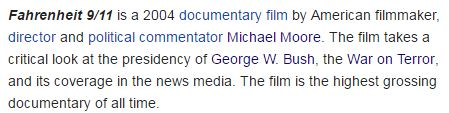They give us 2 release dates for this propaganda piece

5/17/2004 - 5+1+7+2+0+0+4 = 19 (this date could also be written 17/5 a lot like Flight 175)
6/25/2004 - 6+2+5+2+0+0+4 = 19, in 2004 George Bush celebrated his birthday on a date with 19 numerology as well (7 + 6 + 2+0+0+4 = 19)
Including the end date these dates are 1 month and 9 days apart (There were supposedly 19 hijackers on September 11th)Forty = 6+60+90+200+700 = 1056 (English Extended)
Drop the zero from 1056 and you find 911 encoded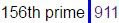From 9/11/2001 to 6/25/2004 is 1019 days - Twenty fifth of June = 20+23+5+14+20+25 + 6+9+6+20+8 + 15+6 + 10+21+14+5 = 227 (English Ordinal)If we measure from 5/17/2004 to Bush's next birthday it gives us 1 month and 19 days - 50 days - Fahrenheit = 3+8+1+9+4+4+1+4+9+7 = 50 (Reverse Reduction)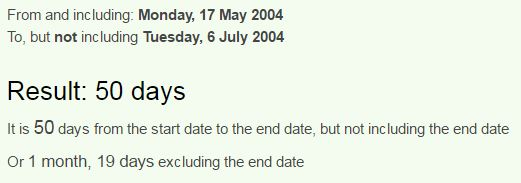Also if we measure from Bush's birthday in 2003 we find 11 months and 19 days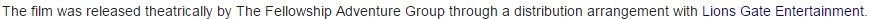Lionsgate = 20+9+50+40+90+7+1+100+5 = 322 (Jewish)
George Bush is born on "Sixth of July" = 100+9+600+200+8 + 60+6 + 10+300+30+700 = 2023 (English Extended)

Lionsgate entertainment = 3+9+6+5+10+7+1+2+5 + 5+5+2+5+9+2+1+9+5+4+5+5+2 = 107 (Single Reduction)
George Bush = 7+5+15+18+7+5 + 2+21+19+8 = 107 (English Ordinal)
Bush declared War on Afghanistan on 10/7/2001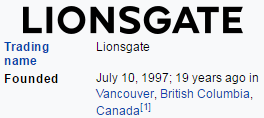Even Lionsgate was founded on a date that would be written 10/7 in most parts of the world.Everywhere they say that Bush spent about 20 minutes in the classroom - "twenty" = 20+23+5+14+20+25 = 107 (English Ordinal)

Documentary was produced by Michael = 4+9+3+8+1+5+3 = 3(Full Reduction)Moore = 13+15+15+18+5 = 66 (English Ordinal)
Thirty three = 2+8+9+9+2+7 + 2+8+9+5+5 = 66 (Full Reduction)

"Michael Moore" = 13+9+3+8+1+5+12 + 13+15+15+18+5 = 117 (English Ordinal)Bush became 46th governor of Texas on 1/17 and he won the presidential election on 11/7/2000 exactly 303 weeks later (When you say September eleventh you are really saying 7/11)I can get into a lot more stuff but a lot of it is gonna be covered in Derek's 9/11 video that's coming soon